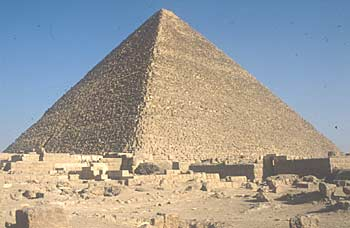A# pyramidA pyramid is a polyhedron whose base is a polygon and whose other faces are triangles that meet at a common vertex, sometimes called the apex. A right pyramid has its apex directly above the center of its base. A square-based pyramid, like the pyramids in Egypt, has a square base and four triangular sides. A triangular pyramid, or tetrahedron, is made from four triangular sides; if regular, it is one of the Platonic solids. The volume of a pyramid is 1/3Ab h, where Ab is the area of the base and h is the perpendicular height of the apex above the base; the surface area is ps, where p is the base perimeter and s is the slant height.